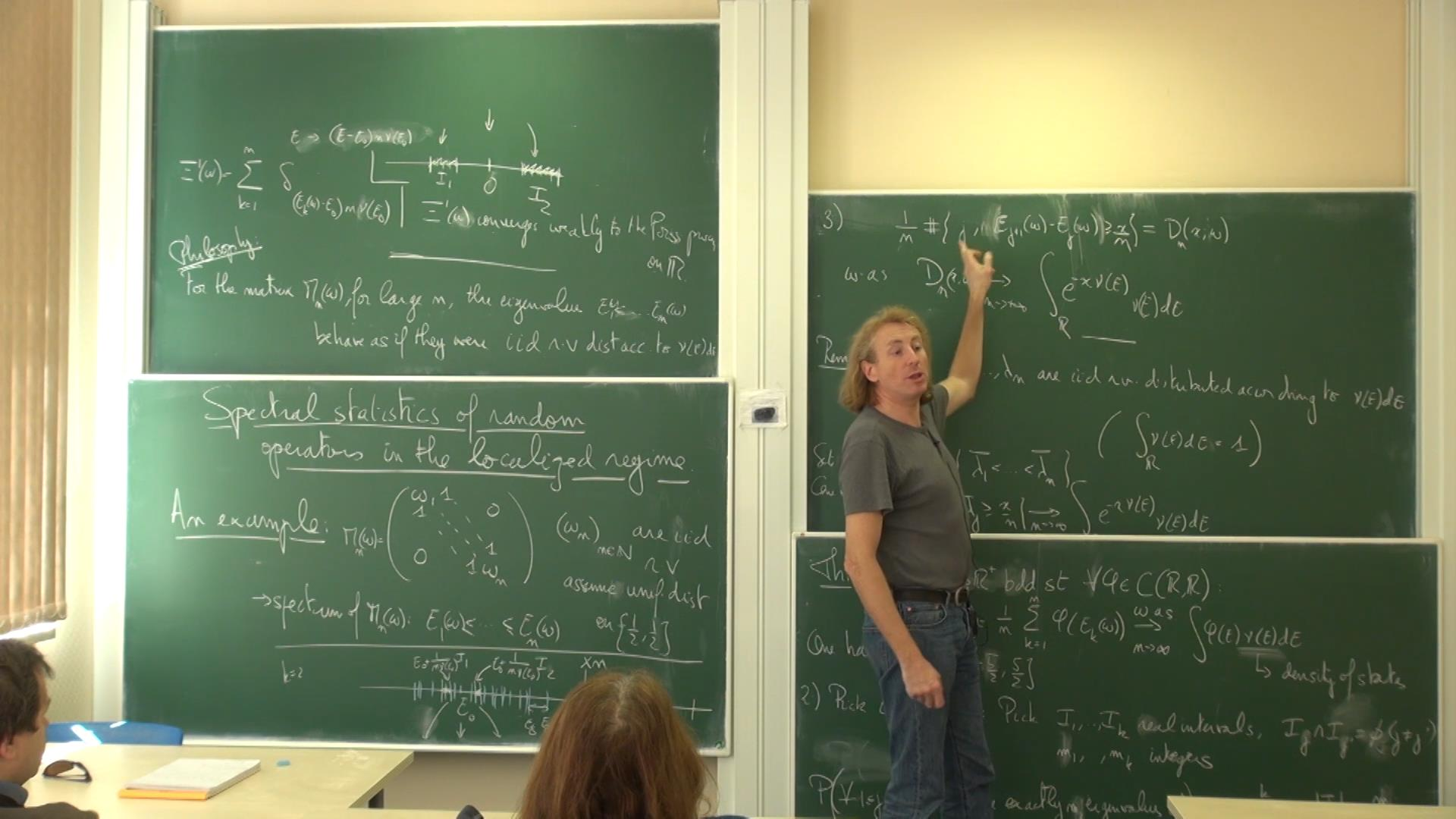# On the statistics of random operators in the localized phase

Курс
Предмет:
Лектор:This set of lectures is intended as a presentation of a number of recent results describing the spectral statistics of random operators in the localized phase.

We will mainly concentrate on the simplest model, the Anderson model, but also discuss more elaborate continuous models. Introduced in condensed matter physics in the late 50s, random Schrödinger operators model the propagation of electrons in a disordered medium. The disordered medium is described through its statistical properties. Ergodicity of the medium ensures an almost sure behavior of the spectrum and the spectral components of the operators : the random families may in a certain respect be considered as a single operator. One basic characteristic of random operators is the existence of a localized phase, i.e., a phase in which multiple backscattering leads to a suppression of propagation. This phase was first studied for random Schr¨odinger operators but then understood to be a characteristic phase for general wave propagation in general random media. Mathematically, this phase corresponds to the presence of intervals of pure point spectrum, i.e., intervals filled with a dense set of eigenvalues and no other spectral type. Moreover, it is typical that, in such a localized interval, the associated eigenfunctions be exponentially decaying.
The aim of the lecture is to describe recent results on the statistics of eigenvalues in the localized phase.

Outline of the lectures:

1. A short introduction to random ergodic operators.

(a) A few models originating in condensed matter physics.
(b) Ergodicity.
(c) The integrated density of states.

1.  Local estimates on eigenvalues.

(a) The Wegner estimate.
(b) The Minami estimate: decorrelation of close by eigenvalues.
(c) General decorrelation estimates.

1. The localize regime.

(a) The phenomenology and meta-theorem.
(b) Multi-scale analysis.
(c) The fractional moment method.

1. Spectral statistics

(a) Local statistics in the bulk of the spectrum
(b) Asymptotic ergodicity and the level spacings distribution in the bulk.
(c) Statistics at the edges of the spectrum.

References:

1. René Carmona and Jean Lacroix. Spectral theory of random Schrödinger operators. Probability and its Applications. Birkhäuser Boston Inc., Boston, MA, 1990.
2. Margherita Disertori, Werner Kirsch, Abel Klein, Frédéric Klopp, and Vincent Rivasseau. Random Schrödinger operators, volume 25 of Panoramas et Synthèses [Panoramas and Syntheses]. Société Mathèmatique de France, Paris, 2008.
3. François Germinet and Frédéric Klopp. Spectral statistics for random Schrödinger operators in the localized regime. ArXiv http://arxiv.org/abs/1011.1832, 2010.
4. François Germinet and Frédéric Klopp. Spectral statistics for the discrete Anderson model in the localized regime. In N. Minami, editor, Spectra of random operators and related topics, 2011. To appear. ArXiv http://arxiv.org/abs/1004.1261.
5. Werner Kirsch. An invitation to random Schrödinger operators. In Random Schrödinger operators, volume 25 of Panor. Synthèses, pages 1–119. Soc. Math. France, Paris, 2008. With an appendix by Frédéric Klopp.
6. Abel Klein. Multiscale analysis and localization of random operators. In Random Schr¨odinger operators, volume 25 of Panor. Synthèses, pages 121–159. Soc. Math. France, Paris, 2008.
7. Frédéric Klopp. Asymptotic ergodicity of the eigenvalues of random operators in the localized phase. ArXiv : http://fr.arxiv.org/abs/1012.0831, 2010.
8. Frédéric Klopp. Decorrelation estimates for the discrete Anderson model. Comm. Math. Phys., 303(1) :233–260, 2011. ArXiv : http://fr.arxiv.org/abs/1004.1261.
9. Frédéric Klopp. Inverse tunneling estimates and applications to the study of spectral statistics of random operators on the real line. ArXiv : http://fr.arxiv.org/abs/1101.0900, 2011.
10. Leonid Pastur and Alexander Figotin. Spectra of random and almostperiodic operators, volume 297 of Grundlehren der Mathematischen Wissenschaften [Fundamental Principles of Mathematical Sciences]. Springer-Verlag, Berlin, 1992.
11. Peter Stollmann. Caught by disorder, volume 20 of Progress in Mathematical Physics. Birkhäuser Boston Inc., Boston, MA, 2001. Bound states in random media.

10